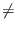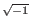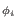Next: Proof that Algorithm Increases Up: Grover's Algorithm Previous: Inversion About Average Operator   Contents

## Proof that Operations are Unitary

If the above matrices not unitary, they will not be physically realizable, at least for systems with time independent Hamiltonians, which are the only ones being considered here. It must be shown that each of the above operations is unitary. As a reminder, a unitary matrix is one whose inverse is the same as the transpose of its complex conjugate, and unitary matrices represent reversible operators that preserve normalization.

The Walsh-Hadamard transformation is one of the fundamental unitary transformations used in quantum computing. The proof is simply a great deal of linear algebra, showing W2 = I (since W is real and symmetric) and is omitted for brevity.

The rotation matrix R with Rij = 0 if ij, and Rii = e. Hereis an arbitrary real number.

It is easy to see R's complex conjugate transposed is the inverse of R. When R is multiplied by it's complex conjugate, the only non-zero elements are on the diagonal, and when the diagonal elements are multiplied the powers of e will cancel, resulting in e0 = 1 on the diagonal, the identity matrix. [Grover96]

To show the inversion about average matrix A is unitary, recall that A may be written as A = - I + 2P where:

• I is the identity matrix
• P is the matrix with each element is equal to 1/N

Recall that P2 = P.

A is real and symmetric, so A is its own transposed complex conjugate, and we must show A2 = I.

A2 = (- I + 2P)2 = I2 -2P - 2P + 4P2 = I - 4P + 4P = I

[Grover96]Next: Proof that Algorithm Increases Up: Grover's Algorithm Previous: Inversion About Average Operator   Contents
Matthew Hayward - Quantum Computing and Grover's Algorithm GitHub Repository